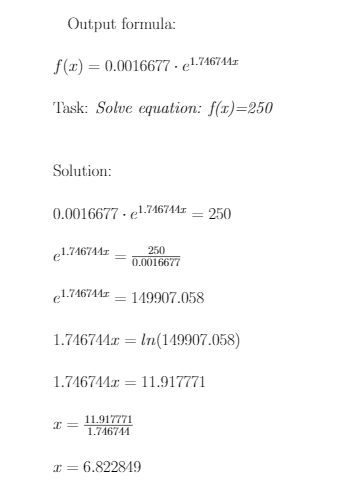# Incorrect trend line plot [v7.1.5.2 x64 Windows]

HI everyone! I need to find x coordiante of the point where y = 250 using exponential trend line function. Given formula by the Libreoffice Calc, we can find that the actual x coordianate should be roughly 6.8, but on the screenshot we can see that the trend line (red color) equals 250 in the point 55-ish which isn’t match with what we found using equations. Given this information I believe that there’s problem with trend line representaion.What could cause this problem? Is there a problem or I just can understand smth? Thank you for an answer in advance.

Which type of chart are you using? Is it X-Y chart, or are your “5”, “10” etc. are treated as names, as suggested by the “strange” non-uniform X axis scale (normal in case of other chart types)?

Wow, numbers were treated as text indeed. Thank you. Now everyting is fine!